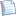# Review 6: Dynamic programmingprintable version

R6:       

### Problem R6.1

Consider the following recursive algorithm.

```public static int f(int n, int r) {     if(r == 0 || r >= n) return 1;     else                 return f(n - 1, r - 1) + f(n - 1, r); } ```

Using dynamic programming, convert this into a method that takes O(n ⋅ r) time.